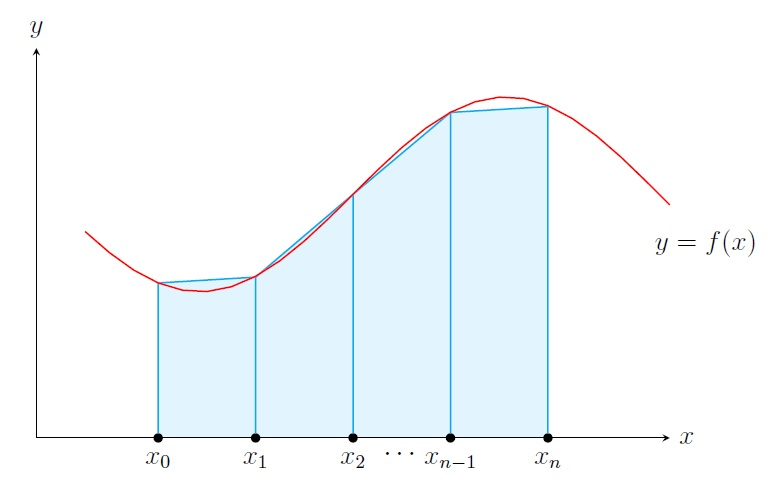Calculus II Home

## Numerical Integration

Sometimes it is hard to calculate a definite integral analytically. For example, $$\displaystyle \int_{0}^1 e^{x^2} \,dx$$. To approximate such an integral we break $$[a,b]$$ into $$n$$ subintervals $$[x_0,x_1],[x_1,x_2],\ldots,[x_{n-1},x_n]$$ where $$x_i=a+i \Delta x$$ and $$\Delta x=(b-a)/n$$. Then we approximate the integral by a finite sum given by a quadrature formula: $\int_{a}^{b} f(x) \,dx \approx \Delta x\sum_{i=1}^n c_if(x_i^*) \text{ for some } c_i \text{ where } x_i^*\in [x_{i-1},x_i]$The Midpoint Rule is $\int_{a}^{b} f(x) \,dx \approx \Delta x \sum_{i=1}^n f\left(\frac{x_{i-1}+x_i}{2} \right).$ Note that the RHS is the sum of areas of $$n$$ rectangles on subintervals $$[x_0,x_1],[x_1,x_2],\ldots,[x_{n-1},x_n]$$ where the height of each rectangle is the height of the curve at the midpoint of the corresponding subinterval.

The Trapezoidal Rule is $\int_{a}^{b} f(x) \,dx \approx \displaystyle\frac{\Delta x}{2} \Big[ f(x_0)+2f(x_1)+\cdots+2f(x_{n-1})+f(x_n) \Big].$ Note that the RHS is the sum of areas of $$n$$ trapezoids on subintervals $$[x_0,x_1],[x_1,x_2],\ldots,[x_{n-1},x_n]$$.

Example. Approximate $$\displaystyle\int_{0}^2 e^x\,dx$$ using $$4$$ subintervals in (a) Trapezoidal Rule, (b) Midpoint Rule.
Solution. First of all let's find the exact integral: $\displaystyle\int_{0}^2 e^x\,dx= e^x \Big]_0^2=e^2-1 \approx 6.389.$ $$n=4\implies h=(2-0)/4=0.5$$ and the 4 subintervals are $$[0,0.5],\; [0.5,1],\; [1,1.5],\; [1.5,2]$$. \begin{align*} \text{Trapezoidal Rule}:\; \int_{0}^2 e^x\,dx &\approx \frac{0.5}{2}\Big[ e^0+2e^{0.5}+2e^{1}+2e^{1.5}+e^{2} \Big]=6.52\\ \text{Midpoint Rule}:\; \int_{0}^2 e^x\,dx &\approx 0.5\Big[ e^{0.25}+e^{0.75}+e^{1.25}+e^{1.75} \Big]=6.32\\ \end{align*}

Last edited